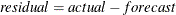### OUT= Data Set

The FORECAST procedure writes the forecast to the output data set named by the OUT= option. The OUT= data set contains the following variables:

• the BY variables

• _TYPE_, a character variable that identifies the type of observation

• _LEAD_, a numeric variable that indicates the number of steps ahead in the forecast. The value of _LEAD_ is 0 for the one-step-ahead forecasts before the start of the forecast period.

• the ID statement variables

• the VAR statement variables, which contain the result values as indicated by the _TYPE_ variable value for the observation

The FORECAST procedure processes each of the input variables listed in the VAR statement and writes several observations for each forecast period to the OUT= data set. The observations are identified by the value of the _TYPE_ variable. The options OUTACTUAL, OUTALL, OUTLIMIT, OUTRESID, OUT1STEP, OUTFULL, and OUTSTD control which types of observations are included in the OUT= data set.

The values of the variable _TYPE_ are as follows:

ACTUAL

The VAR statement variables contain actual values from the input data set. The OUTACTUAL option writes the actual values. By default, only the observations for the forecast period are output.

FORECAST

The VAR statement variables contain forecast values. The OUT1STEP option writes the one-step-ahead predicted values for the observations used to fit the model.

RESIDUAL

The VAR statement variables contain residuals. The residuals are computed by subtracting the forecast value from the actual value (). The OUTRESID option writes observations for the residuals.

Lnn

The VAR statement variables contain lower nn % confidence limits for the forecast values for the future observations specified by the LEAD= option. The value of nn depends on the ALPHA= option; with the default ALPHA=0.05, the _TYPE_ value is L95 for the lower confidence limit observations. The OUTLIMIT option writes observations for the upper and lower confidence limits.

Unn

The VAR statement variables contain upper nn % confidence limits for the forecast values for the future observations specified by the LEAD= option. The value of nn depends on the ALPHA= option; with the default ALPHA=0.05, the _TYPE_ value is U95 for the upper confidence limit observations. The OUTLIMIT option writes observations for the upper and lower confidence limits.

STD

The VAR statement variables contain standard errors of the forecast values. The OUTSTD option writes observations for the standard errors of the forecast.

If no output control options are specified, PROC FORECAST outputs only the forecast values for the forecast periods.

The _TYPE_ variable can be used to subset the OUT= data set. For example, the following data step splits the OUT= data set into two data sets, one that contains the forecast series and the other that contains the residual series. For example

```   proc forecast out=out outresid ...;
...
run;

data fore resid;
set out;
if _TYPE_='FORECAST' then output fore;
if _TYPE_='RESIDUAL' then output resid;
run;
```

See Chapter 3: Working with Time Series Data, for more information about processing time series data sets in this format.